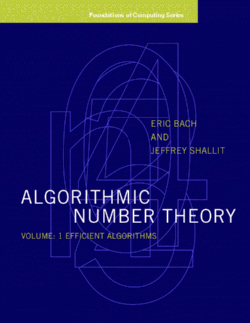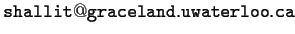# Algorithmic Number TheoryFrom the Preface:

```     This is the first volume of a projected two-volume set on
algorithmic number theory, the design and analysis of algorithms for
problems from the theory of numbers.  This volume focuses primarily on
those problems from number theory that admit relatively efficient
solutions.  The second volume will largely focus on problems for which
efficient algorithms are not known, and applications thereof.

We hope that the material in this book will be useful for readers
at many levels, from the beginning graduate student to experts in the
area.  The early chapters assume that the reader is familiar with the
topics in an undergraduate algebra course:  groups, rings, and fields.
Later chapters assume some familiarity with Galois theory.

As stated above, this book discusses the current state of the art
in algorithmic number theory.  This book is not an elementary
number theory textbook, and so we frequently do not give detailed
proofs of results whose central focus is not computational.  Choosing
otherwise would have made this book twice as long.

However, we firmly believe that a mere list of proved theorems is
not particularly enlightening or useful.  Therefore, we endeavor to
prove as much as is feasible.  Some proofs are left to the reader as
exercises, with outlines suggested in Appendix A.  And every theorem
which is not proved in the text or left as an exercise has a reference
in the "Notes" section that appears at the end of each chapter.

This book is also not intended solely as a practical guide for
those who wish to implement number-theoretic algorithms.  The
variety of architectures of modern machines, and the profusion of
languages and operating systems, frequently make specific remarks on
running times useless.  On the other hand, computational theory without
the influence of practice seems artificial.  Thus, the "Notes"
section of each chapter contains remarks on practical implementations
of the algorithms discussed.

In writing this book, we have found many opportunities to
extend or refine known results.  This is particularly true with
regard to algorithms, many of whose running times were not completely
worked out in the literature.  As an alternative to listing all such
improvements (which is clearly impractical), we give references to
earlier results, such as are known to us, in the appropriate places.
```
The book contains a large bibliography with references to more than 1800 papers and books. The BibTeX files for the bibliography of the book are available for your use without charge.

#### Table of Contents:

```Preface

1. Introduction
1.1. Number theory and complexity.
1.2. Number theory and computation: a brief history.
1.3. Condensed history of the theory of computation.
1.4. Notes on Chapter 1

2. Fundamentals of Number Theory
2.1. Notation, definitions, and some computational problems.
2.2. More definitions.
2.3. Multiplicative functions and M\"obius inversion.
2.4. Notation: Big-O, Little-o, Big-Omega, Big-Theta.
2.5. Abel's identity and Euler's summation formula.
2.6. Asymptotic integration.
2.7. Estimating sums over primes.
2.8. Basic concepts of abstract algebra.
2.8.1. Semigroups, monoids, and groups.
2.8.2. Rings.
2.8.3. Fields.
2.9. Exercises.
2.10. Notes on Chapter 2.

3. A Survey of Complexity Theory.
3.1. Notation.
3.2. The notion of "step".
3.3. Language classes.
3.4. Reductions and NP-completeness.
3.5. Randomized complexity classes.
3.6. A formal computational model.
3.7. Other resources.
3.8. Parallel complexity classes.
3.9. Exercises.
3.10. Notes on Chapter 3.

4. The Greatest Common Divisor.
4.1. The Euclidean algorithm.
4.2. The Euclidean algorithm: worst-case analysis.
4.3. The extended Euclidean algorithm.
4.4. The Euclidean algorithm and continuants.
4.5. Continued fractions.
4.6. The least-remainder Euclidean algorithm.
4.7. The binary gcd algorithm.
4.8. Constructing a gcd-free basis.
4.9. Exercises.
4.10. Notes on Chapter 4.

5. Computing in Z/(n).
5.1. Basics.
5.2. Addition, subtraction, multiplication.
5.3. Multiplicative inverse.
5.4. The power algorithm.
5.5. The Chinese remainder theorem.
5.6. The multiplicative structure of (Z/(n))*
5.7. Quadratic residues.
5.8. The Legendre symbol.
5.9. The Jacobi symbol.
5.10. Exercises.
5.11. Notes on Chapter 5.

6. Finite Fields.
6.1. Basics.
6.2. The Euclidean algorithm.
6.3. Continued fractions.
6.4. Computing in k[X]/f.
6.5. Galois theory.
6.6. The structure of (k[X]/f)*.
6.7. Characters.
6.8. Exercises.
6.9. Notes on Chapter 6.

7. Solving Equations Over Finite Fields.
7.1. Square roots: group-theoretic methods.
7.2. Square roots: field-theoretic methods.
7.3. Computing d-th roots.
7.4. Polynomial factoring algorithms.
7.5. Other results on polynomial factoring.
7.6. Synthesis of finite fields.
7.7. Hensel's lemma.
7.8. Complexity-theoretic results.
7.9. Exercises.
7.10. Notes on Chapter 7.

8. Prime Numbers: Facts and Heuristics.
8.1. Some history.
8.2. The density of primes.
8.3. Sharp estimates and the Riemann hypothesis.
8.4. Primes in arithmetic progressions and the ERH.
8.5. Applications of the ERH.
8.6. Other conjectures about primes.
8.7. Extensions to algebraic numbers.
8.8. Some useful explicit estimates.
8.9. Exercises.
8.10. Notes on Chapter 8.

9. Prime Numbers: Basic Algorithms.
9.1. Primality proofs and Fermat's theorem.
9.2. Primality tests for numbers of special forms.
9.3. Pseudoprimes and Carmichael numbers.
9.4. Probabilistic primality tests.
9.5. ERH-based methods.
9.6. Primality testing using algebraic number theory.
9.7. Generation of "random" primes.
9.8. Prime number sieves.
9.9. Computing pi(x) and p(n).
9.10. Exercises.
9.11. Notes on Chapter 9.

A. Solutions to Exercises.
```
The projected contents of Volume II are given below:
```Chapter 10:  Solving Equations

This chapter will cover algorithms to solve equations of a "Diophantine"
nature.  Examples include linear systems with integer coefficients,
quadratic congruences, expressing integers as sums of squares,
sparse linear systems, lattice algorithms, and applications.

Chapter 11:  Factoring Integers

This chapter will discuss theoretical results about the factorization
of numbers, and present factoring methods based on elementary number
theory.  Such methods include trial division, quadratic residue methods
such as the quadratic sieve, and Pollard's "rho" method.
In addition, it will discuss related methods based on algebraic number
theory, such as class group algorithms and the number field sieve.

Chapter 12:  Discrete Logarithms

This chapter will cover history and algorithms for the discrete logarithm
problem.  Methods include the "baby-step giant-step" method,
the use of group structure, and index calculus methods.  Special
algorithms for finite fields of small characteristic will also be
discussed.

Chapter 13:  Applications of Algebraic Geometry

Many new algorithms in number theory are based on ideas from
algebraic geometry.  After a brief review of algebraic geometry,
this chapter will discuss the elliptic curve factoring method
(and the related p +- 1 methods), primality testing using
elliptic curves, and methods to count points on algebraic
varieties.  It will also discuss ideas for reducing the randomness
requirements of algorithms.

Chapter 14:  Reductions Among Number-Theoretic Problems

This chapter will discuss what is known about the relative computational
complexity of number theoretic problems: for example, what problems are
equivalent in difficulty to factoring?

Chapter 15:  Applications

This chapter will review the new area of "applied number theory".
Examples include the RSA cryptosystem, methods for pseudo-random number
generation, and error-correcting codes.

Chapter 16:   Open Problems

We will close with a list of open problems in algorithmic number theory.
```

E-mail: